Search

# Vane Shear Test

Posted in Civil Engineering Tests |Email This Post |

The undrained shear strength of soft clays can be determined in a laboratory by a vane shear test. The test can also be conducted in the field on the soil at the bottom of a bore hole. The field test can be performed even without drilling a bore hole by direct penetration of the vane from the ground surface if it is provided with a strong shoe to protect it.

The vane shear test instrument consist of a vertical steel rod with four thin stainless steel blades (or vanes) fixed at its bottom end. The IS: 2720-1980 code recommends that the height, H of the vane should be equal to twice the overall diameter, D. The diameter and the length of the rod are recommended as 2.6 mm and 60 mm respectively.

For conducting the test in the laboratory, a specimen of the size 38 mm diameter and 75 mm heightis taken in a container which is fixed securely to the base. The vane is gradually lowered into the specimen till the top of the vane is at a depth of 10 to 20 mm below the top of the specimen. The readings of the strain indicator and torque indicator in the apparatus are noted.

Torque is applied gradually to the upper end of the rod at the rate of about 6 per minute (i.e. 0.1 per second). The torque acting on the specimen is indicated by a pointer fixed to the spring. The torque is applied till the soil specimen fails in shear. The shear strength of the soil is determined using the formula given as below:

Derivation of formula
In the derivation of the formula, it is assumed that the shear strength (s) of the soil is constant on the cylindrical sheared surface and at the top and bottom faces ofthe sheared cylinder. The torque applied (T) must be equal to the sum of the resisting torque at the sides (T1) and that at the top and bottom (T2). Thus

T=T1+T2

The resisting torque on the sides of apparatus is equal to the resisting force developed on the cylindrical surface of the apparatus multiplied by the radial distance to it.
Thus,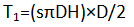The resisting force T2 due to the resisting forces the top and bottom of the sheared cylinder can be determined by the integration ofthe torque developed on a circular ring of radius r and width dr.
Thus,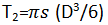From the above equations,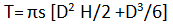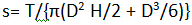For example, if D=1.2 cm, and H=2.4cm, then s=0.158 T

where T is in N-cm and s in N/cm2

The derived equation given above is modified if the top of the vane is above the soil surface and the depth of the vane inside the soil sample is H1. In that case, use the below equation: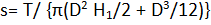The shear strength of the soil sample under undrained conditions will be equal to the apparent cohesion, cu acting on it.

The vane shear test is also used to determine the sensitivity of the soil samples. After the initial tests to determine the undrained shear strength of the soil, the vane is then rotated rapidly through several revolutions to make the soil sample remoulded. The test is then repeated on the remoulded soils samples and the shear strength in remoulded state is determined subsequently. Thus,

Sensitivity (St)= (s) undisturbed/ (s) remoulded

• The test is simple and quick
• Vane shear test is mainly suited the calculation of the in-situ undrained shear strength of non-fissured, fully saturated clay samples.
• Vane shear test can be used to determine the sensitivity of the soil conveniently, if there is a need.